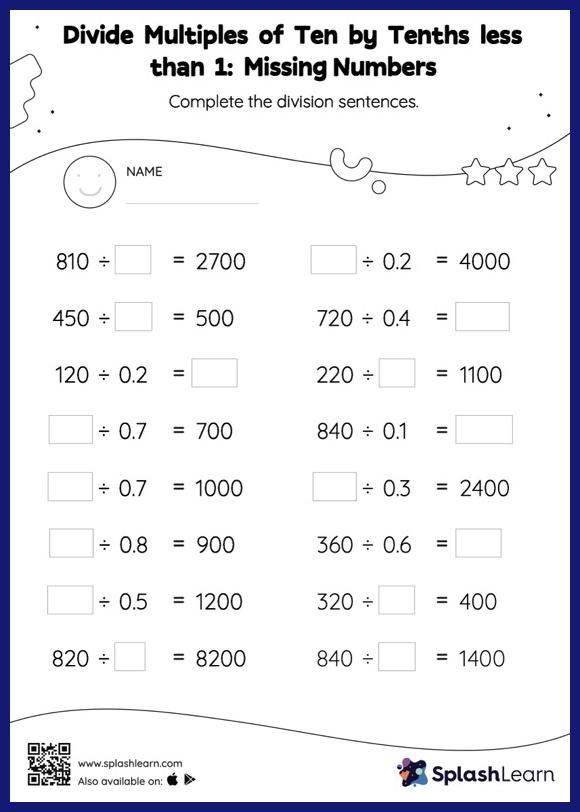# Divide Multiples of Ten by Tenths less than 1 without Remainder: Missing Numbers Worksheet

Home > Divide Multiples of Ten by Tenths less than 1 without Remainder: Missing NumbersThis divide multiples of ten by tenths less than 1 without remainder worksheet consists of tasks to help your young mathematician develop fluency with division. When dividing a number by a decimal, students divide without considering the decimal point and then move each digit of the quotient to the left based on the number of decimal digits in the divisor. Divide multiples of ten by tenths less than 1 without remainder provides plenty of practice with this concept as they find the missing number in decimal division. In each problem, the numbers are laid out in the horizontal format. Students should try to use different strategies involving composing and decomposing numbers to solve these problems. This will help them develop flexibility and fluency.